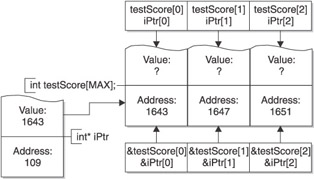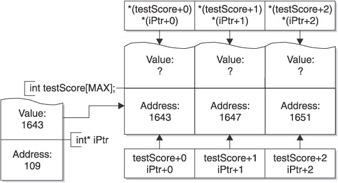# Pointer Arithmetic

The value of a pointer, even though it is an address, is a numeric value. Therefore, you can perform arithmetic operations on a pointer just as you can a numeric value.

### Using a Variable Pointer to Point to an Array

Pointer arithmetic is done often with arrays. However, since you cannot change the value of the name of an array, it being a constant pointer, you first should declare a variable pointer and then assign it to the address of an array.

So, we begin with an established point of reference, let s start with the following program, which outputs the address and value at each element of an array using the name of the array:

` #include <iostream> using namespace std; const int MAX = 3; int main () {  int testScore[MAX] = {4, 7, 1};  for (int i = 0; i < MAX; i++)  {  cout << "The address of index " << i  << " of the array is "<< &testScore[i] << endl;  cout << "The value at index " << i  << " of the array is "<< testScore[i] << endl;  }  return 0; } `

The resulting output is

` The address of index 0 of the array is 0012FECC The value at index 0 of the array is 4 The address of index 1 of the array is 0012FED0 The value at index 1 of the array is 7 The address of index 2 of the array is 0012FED4 The value at index 2 of the array is 1 `

This program used the name of the array, testScore, to access, by index, each element of the array. The name of the array is a constant pointer. The following program modifies the previous program by using a variable pointer, iPtr, to access by index each element of the array.

` #include <iostream> using namespace std; const int MAX = 3; int main () {  int testScore[MAX] = {4, 7, 1};  int* iPtr = testScore;  for (int i = 0; i < MAX; i++)  {  cout << "The address of index " << i  << " of the array is "<< & iPtr[i] << endl;  cout << "The value at index " << i  << " of the array is "<< iPtr[i] << endl;  }  return 0; } `

The following statement in this program sets the variable pointer iPtr to point to the same address as the array name testScore:

` int* iPtr = testScore; `

The array name is not preceded with the address operator (&) because the array name already is an address, namely, the base address of the array. Therefore, after this assignment, iPtr and testScore both point to the beginning of the array. Accordingly, as shown in Figure 11-2, iPtr and testScore have the same value.Figure 11-2: Variable and constant pointers used to access array elements

### Incrementing a Pointer

An important reason for declaring a variable pointer so it points to the same address as the array name is so the variable pointer can be incremented, unlike the array name which cannot be incremented because it is a constant pointer. The following program increments the variable pointer to access each succeeding element of the array:

` #include <iostream> using namespace std; const int MAX = 3; int main () {  int testScore[MAX] = {4, 7, 1};  int* iPtr = testScore;  for (int i = 0; i < MAX; i++, iPtr++)  {  cout << "The address of index " << i  << " of the array is "<< iPtr << endl;  cout << "The value at index " << i  << " of the array is "<< *iPtr << endl;  }  return 0; } `

Incrementing an integer variable increases its value by 1. However, incrementing a pointer variable increases its value by the number of bytes of its data type. This is an example of pointer arithmetic. When you run this program, the first address outputted is 0012FECC, the second 0012FED0, and the third 0012FED4. These hexadecimal addresses are 4 bytes apart because, on the compiler and operating system used by me to run this program, the integer data type takes 4 bytes.

For this reason, as shown in Figure 11-3, iPtr + 1 is not the base address plus 1, but instead is the base address + 4. The same is true of testScore + 1. Consequently, the value at the second element of the array can be expressed one of four ways:

• testScore;

• *(testScore + 1);

• iPtr;

• *(iPtr + 1);Figure 11-3: Effect of incrementing or adding 1 to an address

### Comparing Addresses

Addresses can be compared like any other value. The following program modifies the previous one by incrementing the variable pointer so long as the address to which it points is either less than or equal to the address of the last element of the array, which is & testScore[MAX - 1]:

` #include <iostream> using namespace std; const int MAX = 3; int main () {  int testScore[MAX] = {4, 7, 1};  int* iPtr = testScore;  int i = 0;  while (iPtr <= &testScore[MAX - 1])  {  cout << "The address of index " << i  << " of the array is "<< iPtr << endl;  cout << "The value at index " << i  << " of the array is "<< *iPtr << endl;  iPtr++;  i++;  }  return 0; } `

As Figures 11-2 and 11-3 depict, the comparison to & testScore[MAX - 1] instead could have been made to testScore + MAX “ 1.

### Decrementing a Pointer

The same considerations apply to decrementing a pointer, which decreases its value by the number of bytes of its data type. Decrementing a pointer can be used to step backwards through an array.

` #include <iostream> using namespace std; const int MAX = 3; int main () {  int testScore[MAX] = {4, 7, 1};  int* iPtr = &testScore[MAX - 1];  int i = MAX - 1;  while (iPtr >= &testScore)  {  cout << "The address of index " << i  << " of the array is "<< iPtr << endl;  cout << "The value at index " << i  << " of the array is "<< *iPtr << endl;  iPtr--;  i--;  }  return 0; } `

The output is therefore

` The address of index 2 of the array is 0012FED4 The value at index 2 of the array is 1 The address of index 1 of the array is 0012FED0 The value at index 1 of the array is 7 The address of index 0 of the array is 0012FECC The value at index 0 of the array is 4 `

The key statement is

` int* iPtr = &testScore[MAX - 1]; `

This statement has the variable pointer point to the last address in the array. That address then is decremented in the loop so that the pointer variable points to the preceding address in the array. The loop continues so long as the address pointed to by the pointer variable is not before the base address of the array.

As discussed previously, the pointer variable also could have been initialized as follows :

` int* iPtr = testScore + MAX - 1; `C++ Demystified(c) A Self-Teaching Guide
ISBN: 72253703
EAN: N/A
Year: 2006
Pages: 148

flylib.com © 2008-2017.
If you may any questions please contact us: flylib@qtcs.net